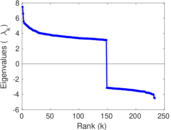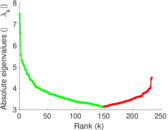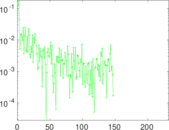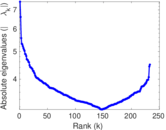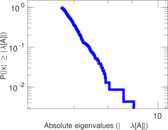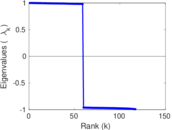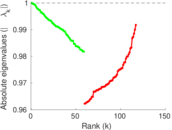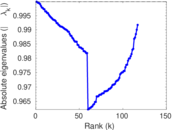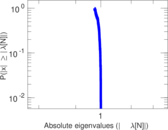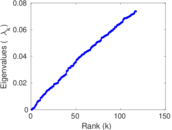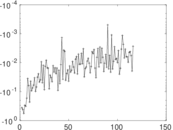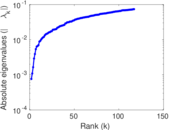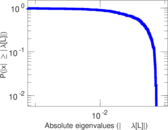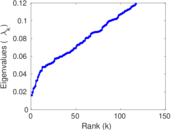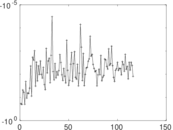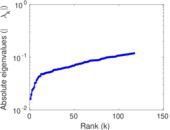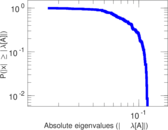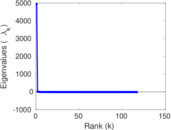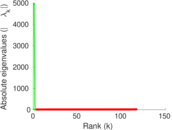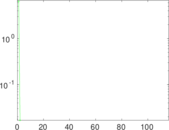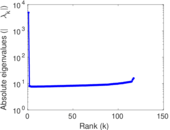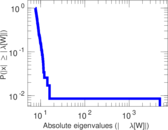US power grid

This undirected network contains information about the power grid of the Western States of the United States of America. An edge represents a power supply line. A node is either a generator, a transformator or a substation.

 Code UG Internal name opsahl-powergrid Name US power grid Data source http://toreopsahl.com/datasets/#uspowergrid AvailabilityDataset is available for download Consistency checkDataset passed all tests Category Infrastructure network Node meaning Node Edge meaning Supply Network format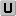Unipartite, undirected Edge typeUnweighted, no multiple edges LoopsDoes not contain loops

Statistics

 Size n = 4,941 Volume m = 6,594 Loop count l = 0 Wedge count s = 18,933 Claw count z = 26,050 Cross count x = 38,357 Triangle count t = 651 Square count q = 979 4-Tour count T4 = 96,752 Maximum degree dmax = 19 Average degree d = 2.669 10 Fill p = 0.000 540 303 Size of LCC N = 4,941 Diameter δ = 46 50-Percentile effective diameter δ0.5 = 19.675 7 90-Percentile effective diameter δ0.9 = 28.172 8 Median distance δM = 20 Mean distance δm = 20.094 1 Gini coefficient G = 0.324 777 Balanced inequality ratio P = 0.379 588 Relative edge distribution entropy Her = 0.978 307 Power law exponent γ = 2.246 78 Tail power law exponent γt = 7.631 00 Tail power law exponent with p γ3 = 7.631 00 p-value p = 0.830 000 Degree assortativity ρ = +0.003 456 99 Degree assortativity p-value pρ = 0.691 396 Clustering coefficient c = 0.103 153 Spectral norm α = 7.483 05 Algebraic connectivity a = 0.000 759 212 Spectral separation |λ1[A] / λ2[A]| = 1.132 21 Non-bipartivity bA = 0.398 772 Normalized non-bipartivity bN = 0.008 259 16 Algebraic non-bipartivity χ = 0.016 118 7 Spectral bipartite frustration bK = 0.001 509 75 Controllability C = 721 Relative controllability Cr = 0.145 922

Plots

Fruchterman–Reingold graph drawing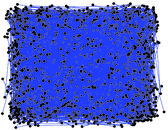Degree distribution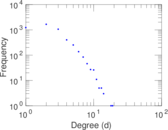Cumulative degree distribution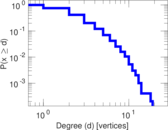Lorenz curve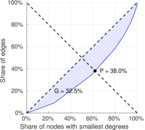Spectral distribution of the adjacency matrix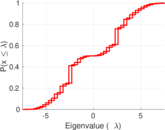Spectral distribution of the normalized adjacency matrix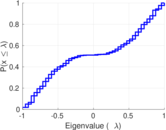Spectral distribution of the Laplacian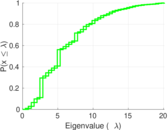Spectral graph drawing based on the adjacency matrix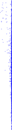Spectral graph drawing based on the Laplacian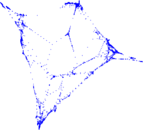Spectral graph drawing based on the normalized adjacency matrixDegree assortativity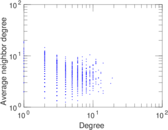Zipf plot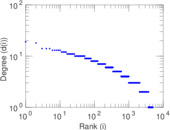Hop distribution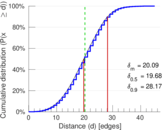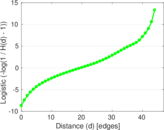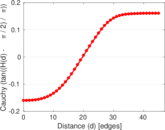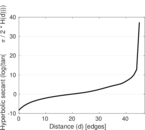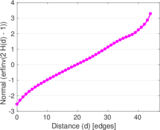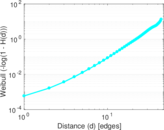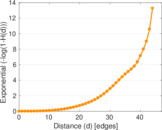Double Laplacian graph drawing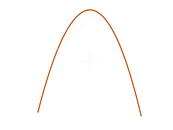Delaunay graph drawing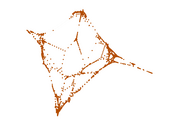Clustering coefficient distribution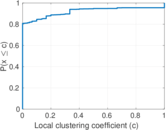Average neighbor degree distribution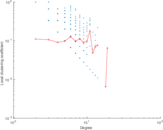SynGraphy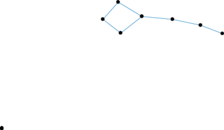Matrix decompositions plots